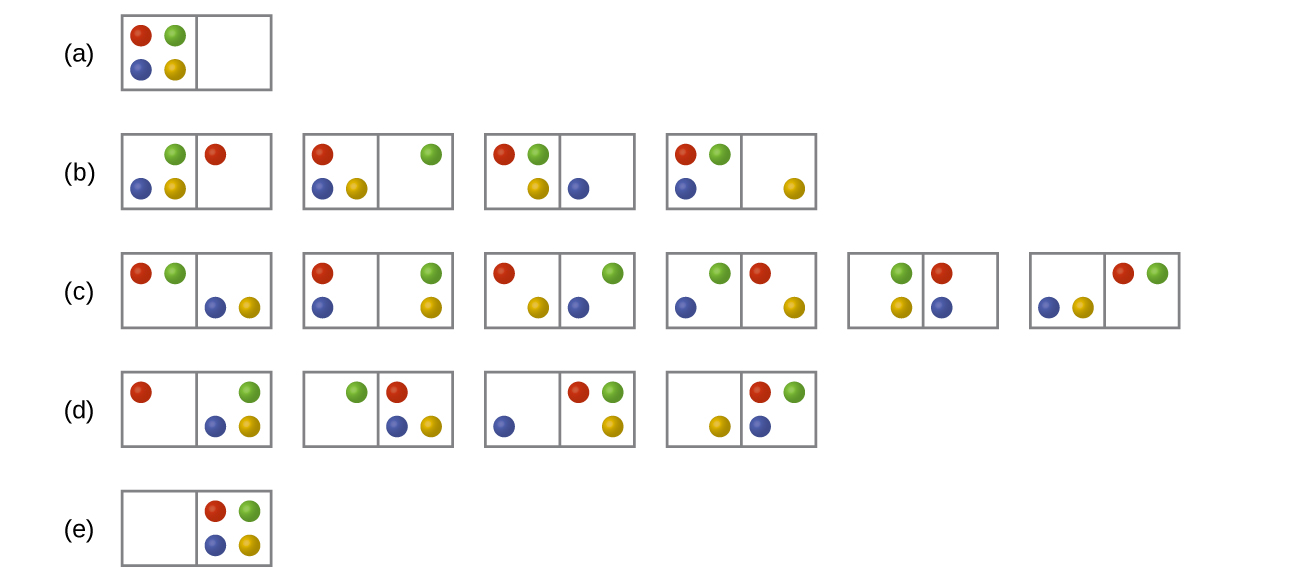Chemistry Practice Problems Boltzmann Equation Practice Problems Solution:  In Figure 16.8 (below) all possible distributions...

⚠️Our tutors found the solution shown to be helpful for the problem you're searching for. We don't have the exact solution yet.

# Solution:  In Figure 16.8 (below) all possible distributions and microstates are shown for four different particles shared between two boxes. Determine the entropy change, ΔS , if the particles are initially evenly distributed between the two boxes, but upon redistribution all end up in Box (b).

###### Problem

In Figure 16.8 (below) all possible distributions and microstates are shown for four different particles shared between two boxes. Determine the entropy change, ΔS , if the particles are initially evenly distributed between the two boxes, but upon redistribution all end up in Box (b).Boltzmann Equation

Boltzmann Equation

#### Q. In the following table shows the possible arrangements of four molecules in a two-bulbed flask. What are the possible arrangements if there is one mol...

Solved • Fri Nov 30 2018 14:01:14 GMT-0500 (EST)

Boltzmann Equation

#### Q. With two dice, each numbered 1-6, there are two possible ways to roll a 3: Thus, for the outcome of 3 (a particular macrostate) there are 2 microstate...

Solved • Mon Apr 02 2018 15:08:10 GMT-0400 (EDT)

Boltzmann Equation

#### Q. With two dice, each numbered 1-6, there are two possible ways to roll a 3. Thus, for the outcome of 3 (a particular macrostate) there are 2 microstate...

Solved • Fri Mar 23 2018 17:48:24 GMT-0400 (EDT)

Boltzmann Equation

#### Q. A negative change in entropy indicates thata) the products have a greater number of available energy microstates than the reactants.b) the products ha...

Solved • Tue Feb 20 2018 12:39:38 GMT-0500 (EST)